# Difference between revisions of "Filter"

dual ideal

A non-empty subsetof a partially ordered setsatisfying the conditions: a) if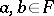and if the infimum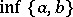exists, then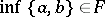; and b) ifand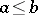, then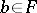. The concept of a filter is dual to that of an ideal of a partially ordered set.

A filter over a non-empty set(or in a set) is a proper filter of the set of subsets of, ordered by inclusion i.e. any non-empty collectionof subsets ofsatisfying the conditions: If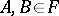, then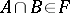; if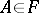and, then; the empty set does not belong to.

A filter base is a system of subsets ofsatisfying the two conditions: 1) the empty set does not belong to it; and 2) the intersection of two subsets belonging to it contains some third subset belonging to it. Every filter is completely determined by any of its filter bases. The system of all subsets ofthat contain some element of a given filter base is a filter. It is said to be spanned by this base.

The set of all filters over a given set is partially ordered by inclusion. A maximal element of it is called an ultrafilter (a maximal proper filter in any Boolean algebra is also called an ultrafilter).

Examples of filters. 1) Let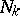be the subset of the natural numbers consisting of those numbers that are multiples of; the system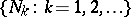is a filter base; the filter spanned by this base consists of those subsets that contain some. 2) The collection of all subsets containing a certain fixed non-empty subset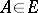is a filter over, called a principal filter. All filters over a finite set are principal. 3) Ifis an infinite set of cardinalityandis the collection of all subsets ofwhose complements have cardinality less than, thenis a filter (called a Fréchet filter). A Fréchet filter is an example of a non-principal filter. 4) The system of subsets containing some fixed point of a set is also a filter; moreover, it is an ultrafilter. 5) Suppose a topology is given on $E$; then the neighbourhoods of any point $x \in E$ (the subsets of $E$ containing $x$ in the interior) form a filter.

How to Cite This Entry:
Filter. Encyclopedia of Mathematics. URL: http://encyclopediaofmath.org/index.php?title=Filter&oldid=13468
This article was adapted from an original article by V.I. MalykhinT.S. Fofanova (originator), which appeared in Encyclopedia of Mathematics - ISBN 1402006098. See original article